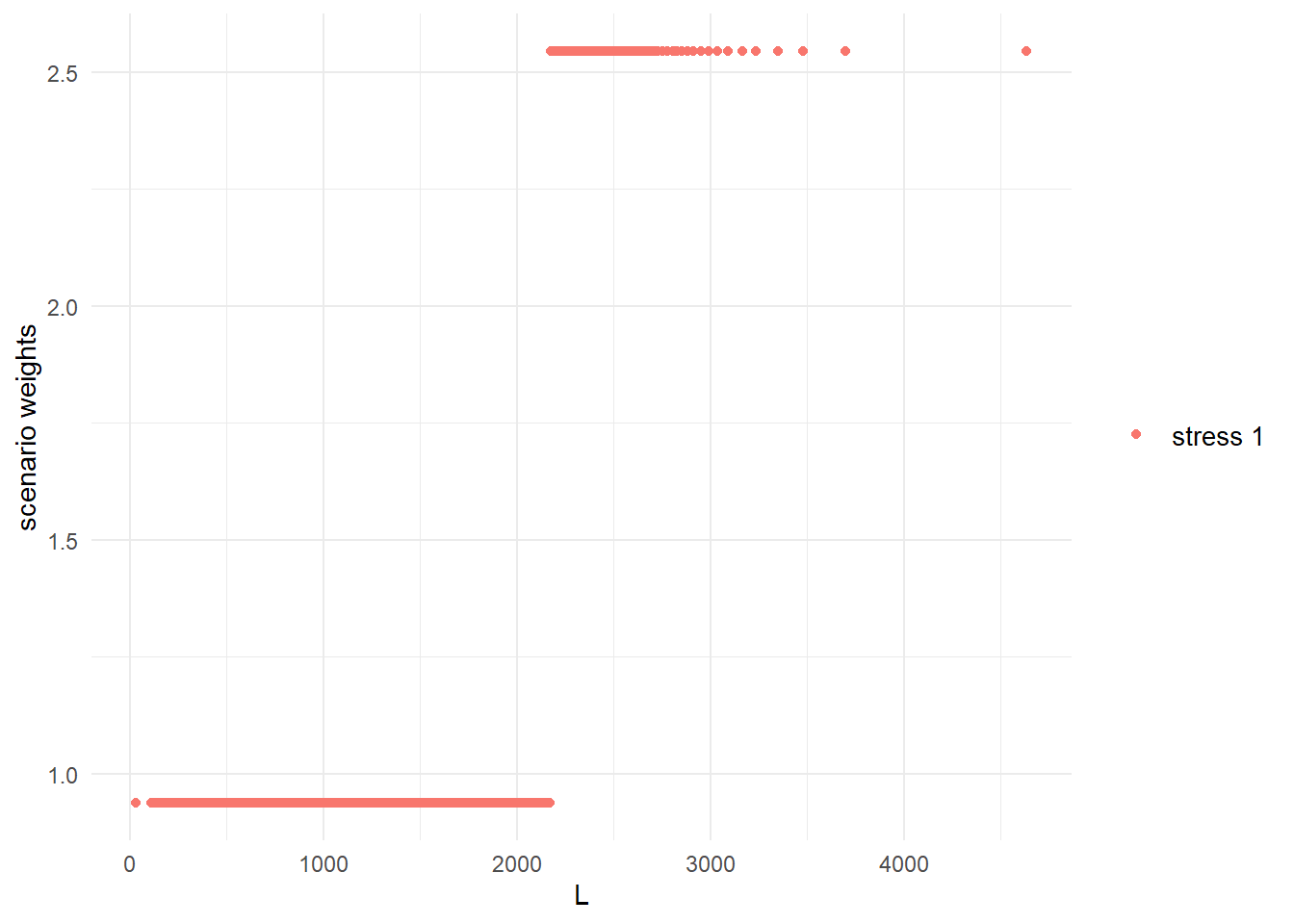# 1 Introduction

## 1.1 Background and contribution

Complex quantitative models are used extensively in actuarial and financial risk management applications, as well as in wider fields such as environmental risk modelling . The complexity of such models (high dimensionality of inputs; non-linear relationships) motivates the performance of sensitivity analyses, with the aim of providing insight into the ways that model inputs interact and impact upon the model output.

When model inputs are subject to uncertainty, global sensitivity methods are often used, considering the full space of (randomly generated) multivariate scenarios, which represent possible configurations of the model input vector. The particular task of ranking the importance of different model inputs leads to the use of sensitivity measures, which assign a score to each model input. A rich literature on global sensitivity analysis exists, with variance decomposition methods being particularly prominent; see and for wide-ranging reviews. The R package sensitivity implements a wide range of sensitivity analysis approaches and measures.

We introduce an alternative approach to sensitivity analysis called Scenario Weights for Importance Measurement (SWIM) and present the R package implementing it . The aim of this paper is to provide an accessible introduction to the concepts underlying SWIM and a vignette demonstrating how the package is used. SWIM quantifies how distorting a particular model component (which could be a model input, output, or an intermediate quantity) impacts all other model components. The SWIM approach can be summarised as follows:

1. The starting point is a table of simulated scenarios, each column containing realisations of a different model component. This table forms the baseline model as well as the dataset on which the SWIM bases its calculations.

2. A stress is defined as a particular modification of a model component (or group of components). This could relate to a change in moments, probabilities of events of interest, or risk measures, such as Value-at-Risk or Expected Shortfall (e.g. ).

3. SWIM calculates a set of scenario weights, acting upon the simulated scenarios and thus modifying the relative probabilities of scenarios occurring. Scenario weights are derived such that the defined stress on model components is fulfilled, while keeping the distortion to the baseline model to a minimum, as quantified by the Kullback-Leibler divergence (relative entropy).

4. Given the calculated scenario weights, the impact of the stress on the distributions of all model components is worked out and sensitivity measures, useful for ranking model components, are evaluated.

A key benefit of SWIM are that it provides a sensitivity analysis framework that is economical both computationally and in terms of the information needed to perform the analysis. Specifically, sensitivity analysis is performed using only one set of simulated scenarios. No further simulations are needed, thus eliminating the need for repeated evaluation of the model, which could be numerically expensive. Furthermore, the user of SWIM needs to know neither the explicit form of the joint distribution of model components nor the exact form of functional relations between them. Hence, SWIM is appropriate for the analysis of black box models, thus having a wide scope of applications.

The SWIM approach is largely based on and uses theoretical results on risk measures and sensitivity measures developed in that paper. An early sensitivity analysis approach based on scenario weighting was proposed by . The Kullback-Leibler divergence has been used extensively in the financial risk management literature – papers that are conceptually close to SWIM include ; ; and . Some foundational results related to the minimisation of the Kullback-Leibler divergence are provided in .

## 1.2 Installation

The SWIM package can be installed from CRAN or through GitHub:

# directly from CRAN
install.packages("SWIM")
# and the development version from GitHub
devtools::install_github("spesenti/SWIM")

## 1.3 Structure of the paper

Section 2 provides an introduction to SWIM, illustrating key concepts and basic functionalities of the package on a simple example. Section 3 contains technical background on the optimisations that underlay the SWIM package implementation. Furthermore, Section 3 includes a brief reference guide, providing an overview of implemented R functions, objects, and graphical/analysis tools. Finally, a detailed case study of a credit risk portfolio is presented in Section 4. Through this case study, advanced capabilities of SWIM for sensitivity analysis are demonstrated.

# 2 What is SWIM?

## 2.1 Sensitivity testing and scenario weights

The purpose of SWIM is to enable sensitivity analysis of models implemented in a Monte Carlo simulation framework, by distorting (stressing) some of the models’ components and monitoring the resulting impact on quantities of interest. To clarify this idea and explain how SWIM works, we first define the terms used. By a model, we mean a set of $$n$$ (typically simulated) realisations from a vector of random variables $$(X_1,\dots,X_d)$$, along with scenario weights $$W$$ assigned to individual realisations, as shown in the table below. Hence each of of the columns 1 to $$d$$ corresponds to a random variable, called a model component, while each row corresponds to a scenario, that is, a state of the world.

Table 2.1: Illustration of the SWIM framework, that is the baseline model, the stressed model and the scenario weights.
$$X_1$$ $$X_2$$ $$\dots$$ $$X_d$$ $$W$$
$$x_{11}$$ $$x_{21}$$ $$\dots$$ $$x_{d1}$$ $$w_1$$
$$x_{12}$$ $$x_{22}$$ $$\dots$$ $$x_{d2}$$ $$w_2$$
$$\vdots$$ $$\vdots$$ $$\ddots$$ $$\vdots$$ $$\vdots$$
$$x_{1n}$$ $$x_{2n}$$ $$\dots$$ $$x_{dn}$$ $$w_n$$

Each scenario has a scenario weight, shown in the last column, such that, scenario $$i$$ has probability $$\frac{w_i}{n}$$ of occurring. Scenario weights are always greater or equal than zero and have an average of 1. When all scenario weights are equal to 1, such that the probability of each scenario is $$\frac 1 n$$ (the standard Monte Carlo framework), we call the model a baseline model – consequently weights of a baseline model will never be explicitly mentioned. When scenario weights are not identically equal to 1, such that some scenarios are more weighted than others, we say that we have a stressed model.

The scenario weights make the joint distribution of model components under the stressed model different, compared to the baseline model. For example, under the baseline model, the expected value of $$X_1$$ and the cumulative distribution function of $$X_1$$, at threshold $$t$$, are respectively given by:

$E(X_1)=\frac 1 n \sum_{i=1}^nx_{1i},\quad F_{X_1}(t)= P(X_1\leq t)=\frac 1 n \sum_{i=1}^n \mathbf 1 _{x_{1i}\leq t},$

where $$\mathbf 1 _{x_{1i}\leq t}=1$$ if $$x_{1i}\leq t$$ and $$0$$ otherwise. For a stressed model with scenario weights $$W$$, the expected value $$E^W$$ and cumulative distribution function $$F^W$$ become:

$E^W(X_1)=\frac 1 n \sum_{i=1}^n w_i x_{1i},\quad F_{X_1}^W(t)=P^W(X_1\leq t)=\frac 1 n \sum_{i=1}^n w_i \mathbf 1 _{x_{1i}\leq t}.$

Similar expressions can be derived for more involved quantities, such as higher (joint) moments and quantiles.

The logic of stressing a model with SWIM then proceeds as follows. An analyst or modeller is supplied with a baseline model, in the form of a matrix of equiprobable simulated scenarios of model components. The modeller wants to investigate the impact of a change in the distribution of, say, $$X_1$$. To this effect, she chooses a stress on the distribution of $$X_i$$, for example requiring that $$E^W(X_1)=m$$; we then say that she is stressing $$X_1$$ and, by extension, the model. Subsequently, SWIM calculates the scenario weights such that the stress is fulfilled and the distortion to the baseline model induced by the stress is as small as possible; specifically the Kullback-Leibler divergence (or relative entropy) between the baseline and stressed models is minimised. (See Section 3.1 for more detail on the different types of possible stresses and the corresponding optimisation problems). Once scenario weights are obtained, they can be used to determine the stressed distribution of any model component or function of model components. For example, for scenario weights $$W$$ obtained through a stress on $$X_1$$, we may calculate

$E^W(X_2)=\frac 1 n\sum_{i=1}^n w_i x_{2i},\quad E^W(X_1^2+X_2^2)=\frac 1 n \sum_{i=1}^n w_i \left(x_{1i}^2+ x_{2i}^2 \right).$

Through this process, the modeller can monitor the impact of the stress on $$X_1$$ on any other random variable of interest. It is notable that this approach does not necessitate generating new simulations from a stochastic model. As the SWIM approach requires a single set of simulated scenarios (the baseline model) it offers a clear computational benefit.

## 2.2 An introductory example

Here, through an example, we illustrate the basic concepts and usage of SWIM for sensitivity analysis. More advanced usage of SWIM and options for constructing stresses are demonstrated in Sections 3 and 4. We consider a simple portfolio model, with the portfolio loss defined by $$Y=Z_1+Z_2+Z_3$$. The random variables $$Z_1,Z_2,Z_3$$ represent normally distributed losses, with $$Z_1\sim N(100,40^2)$$, $$Z_2\sim Z_3\sim N(100,20^2)$$. $$Z_1$$ and $$Z_2$$ are correlated, while $$Z_3$$ is independent of $$(Z_1,Z_2)$$. Our purpose in this example is to investigate how a stress on the loss $$Z_1$$ impacts on the overall portfolio loss $$Y$$. First we derive simulated data from the random vector $$(Z_1,Z_2,Z_3,Y)$$, forming our baseline model.

set.seed(0)
# number of simulated scenarios
n.sim <- 10 ^ 5
# correlation between Z1 and Z2
r <- 0.5
# simulation of Z1  and Z2
# constructed as a combination of independent standard normals U1, U2
U1 <- rnorm(n.sim)
U2 <- rnorm(n.sim)
Z1 <- 100 + 40 * U1
Z2 <- 100 + 20 * (r * U1 + sqrt(1 - r ^ 2) * U2)
# simulation of Z3
Z3 <- rnorm(n.sim, 100, 20)
# portfolio loss Y
Y <- Z1 + Z2 + Z3
# data of baseline model
dat <- data.frame(Z1, Z2, Z3, Y)

Now we introduce a stress to our baseline model. For our first stress, we require that the mean of $$Z_1$$ is increased from 100 to 110. This is done using the stress function, which generates as output the SWIM object str.mean. This object stores the stressed model, i.e. the realisations of the model components and the scenario weights. In the function call, the argument k = 1 indicates that the stress is applied on the first column of dat, that is, on the realisations of the random variable $$Z_1$$.

library(SWIM)
str.mean <- stress(type = "mean", x = dat, k = 1, new_means = 110)
##   cols required_moment achieved_moment abs_error rel_error
## 1    1             110             110     1e-08   9.2e-11
summary(str.mean, base = TRUE)
## $base ## Z1 Z2 Z3 Y ## mean 1.0e+02 99.9404 99.9843 299.9811 ## sd 4.0e+01 19.9969 19.9818 56.6386 ## skewness -6.1e-04 0.0012 -0.0025 -0.0023 ## ex kurtosis -1.1e-02 -0.0089 -0.0125 -0.0093 ## 1st Qu. 7.3e+01 86.4745 86.4816 261.6121 ## Median 1.0e+02 99.9866 100.0091 300.0548 ## 3rd Qu. 1.3e+02 113.3957 113.4934 338.2670 ## ##$stress 1
##                   Z1       Z2       Z3        Y
## mean        110.0000 102.4437  99.9828 312.4265
## sd           40.0331  19.9953  19.9761  56.6170
## skewness     -0.0024  -0.0015  -0.0049  -0.0037
## ex kurtosis  -0.0050  -0.0031  -0.0154  -0.0011
## 1st Qu.      82.9984  88.9771  86.4815 274.2200
## Median      110.0759 102.4810  99.9954 312.5039
## 3rd Qu.     136.9310 115.8744 113.5019 350.6120

The summary function, applied to the SWIM object str.mean, shows how the distributional characteristics of all random variables change from the baseline to the stressed model. In particular, we see that the mean of $$Z_1$$ changes to its required value, while the mean of $$Y$$ also increases. Furthermore there is a small impact on $$Z_2$$, due to its positive correlation to $$Z_1$$.

Beyond considering the standard statistics evaluated via the summary function, stressed probability distributions can be plotted. In Figure 2.1 we show the impact of the stress on the cumulative distribution functions (cdf) of $$Z_1$$ and $$Y$$. It is seen how the stressed cdfs are lower than the original (baseline) ones. Loosely speaking, this demonstrates that the stress has increased (in a stochastic sense) both random variables $$Z_1$$ and $$Y$$. While the stress was on $$Z_1$$, the impact on the distribution of the portfolio $$Y$$ is clearly visible.

# refer to variable of interest by name...
plot_cdf(str.mean, xCol = "Z1", base = TRUE)
# ... or column number
plot_cdf(str.mean, xCol = 4, base = TRUE)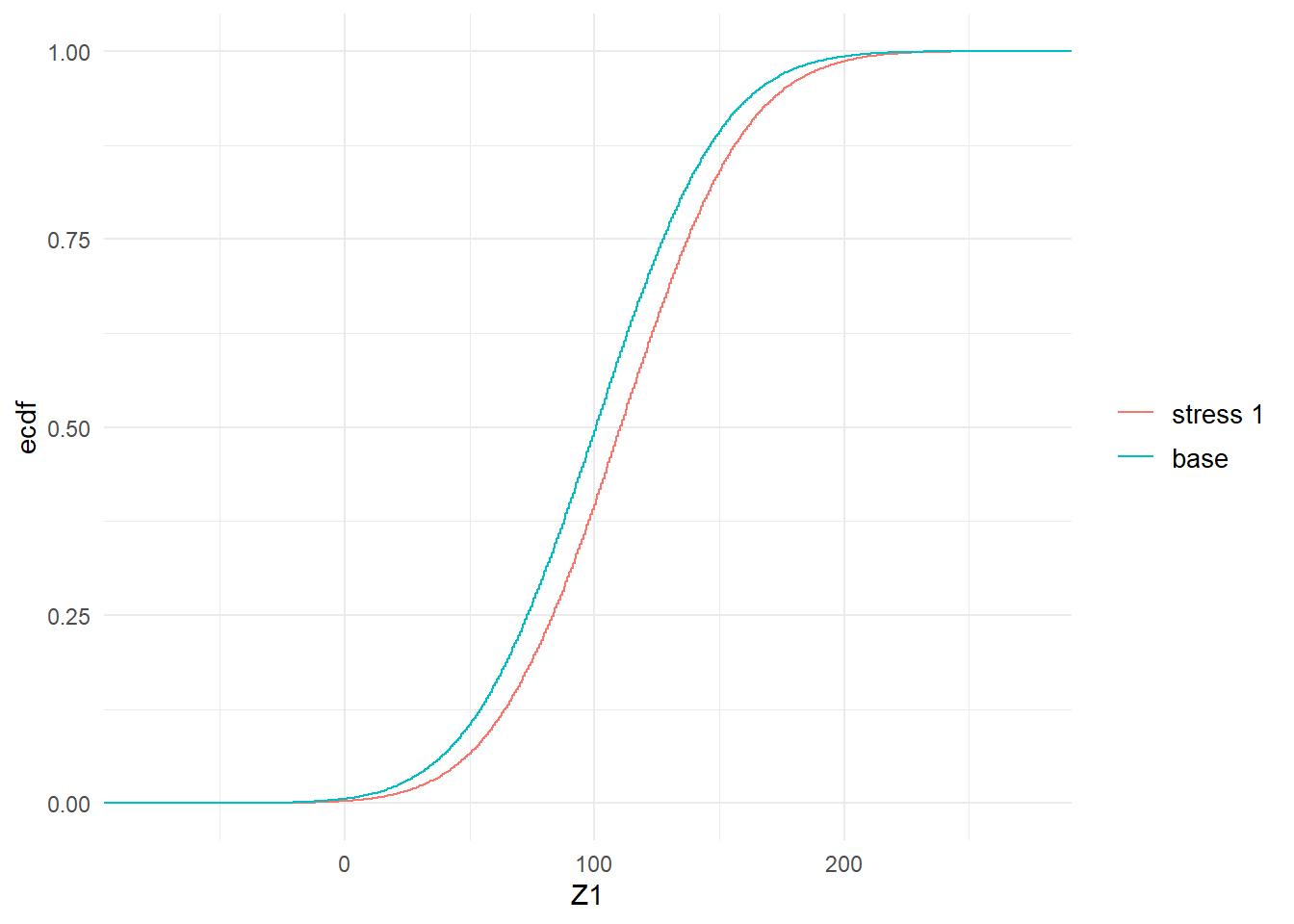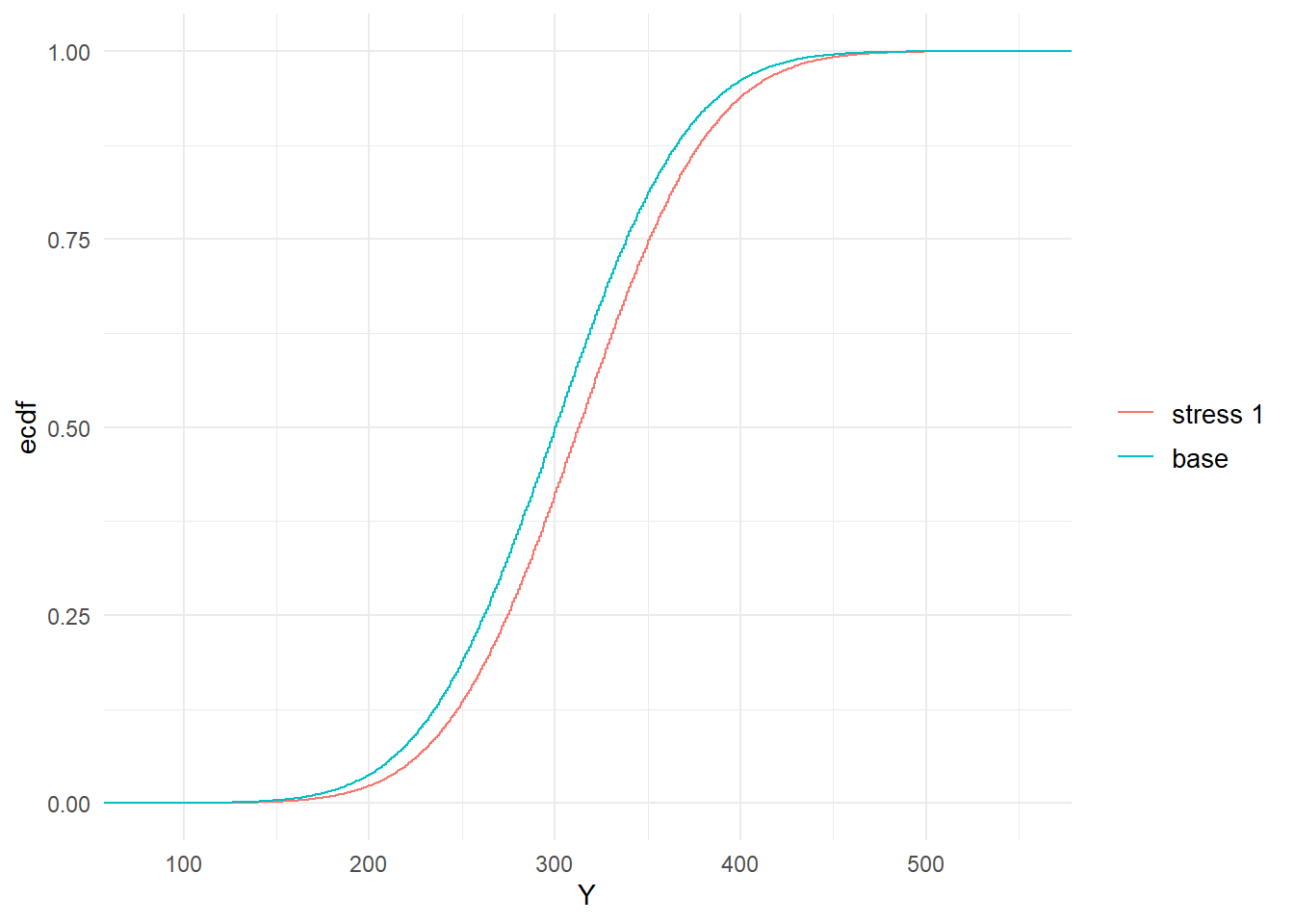Figure 2.1: Baseline and stressed empirical distribution functions of model components $$Z_1$$ (left) and $$Y$$ (right), subject to a stress on the mean of $$Z_1$$.

The scenario weights, given their central role, can be extracted from a SWIM object. In Figure 2.2, the scenario weights from str.mean are plotted against realisations from $$Z_1$$ and $$Y$$ respectively. It is seen how the weights are increasing in the realisations from $$Z_1$$. This is a consequence of the weights’ derivation via a stress on the model component $$Z_1$$. The increasingness shows that those scenarios for which $$Z_1$$ is largest are assigned a higher weight. The relation between scenario weights and $$Y$$ is still increasing (reflecting that high outcomes of $$Y$$ tend to receive higher weights), but no longer deterministic (showing that $$Y$$ is not completely driven by changes in $$Z_1$$).

# parameter n specifies the number of scenario weights plotted
plot_weights(str.mean, xCol = "Z1", n = 1000)
# specifying the limits of the x-axis
plot_weights(str.mean, xCol = "Y", x_limits = c(90, 550), n = 1000)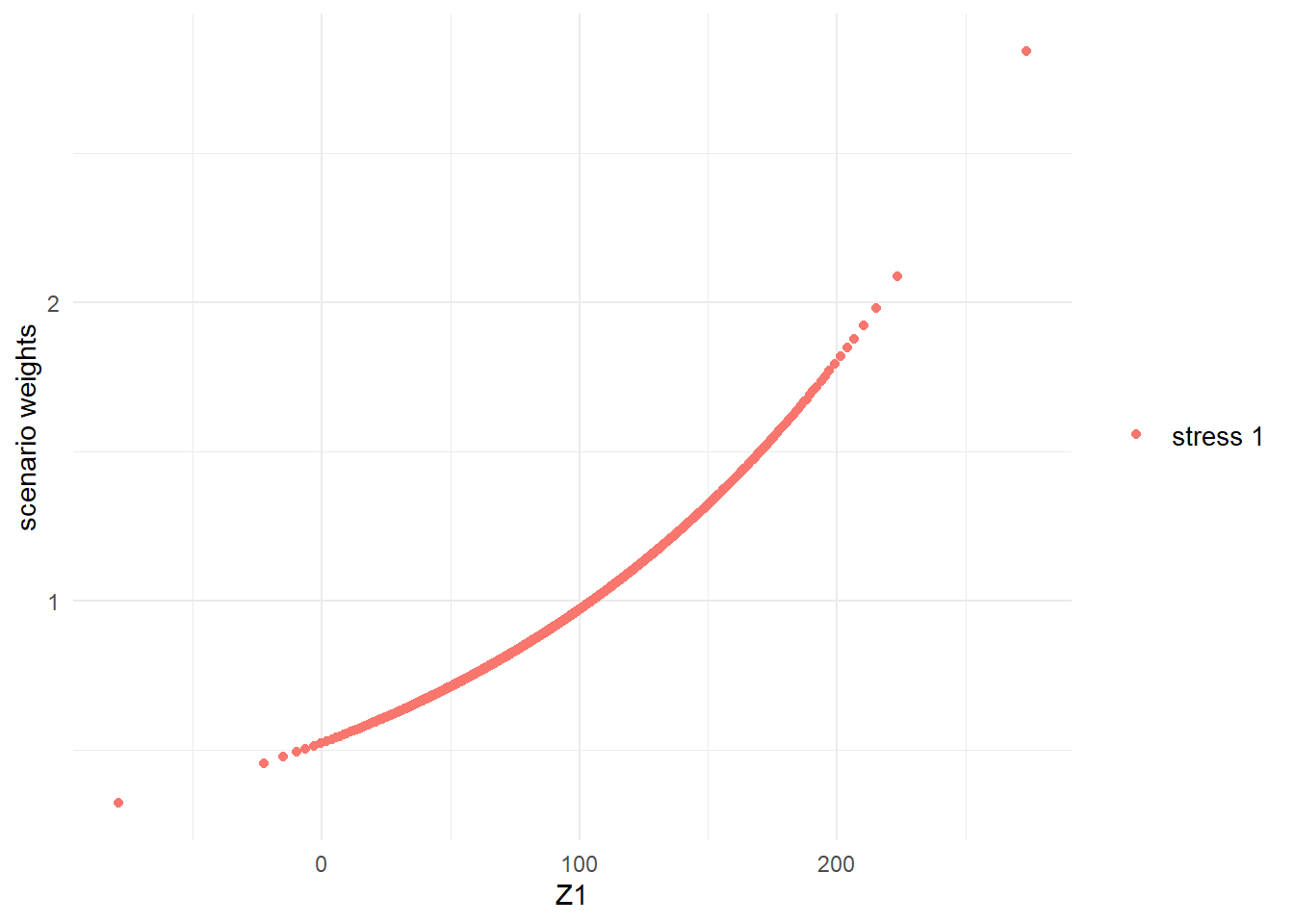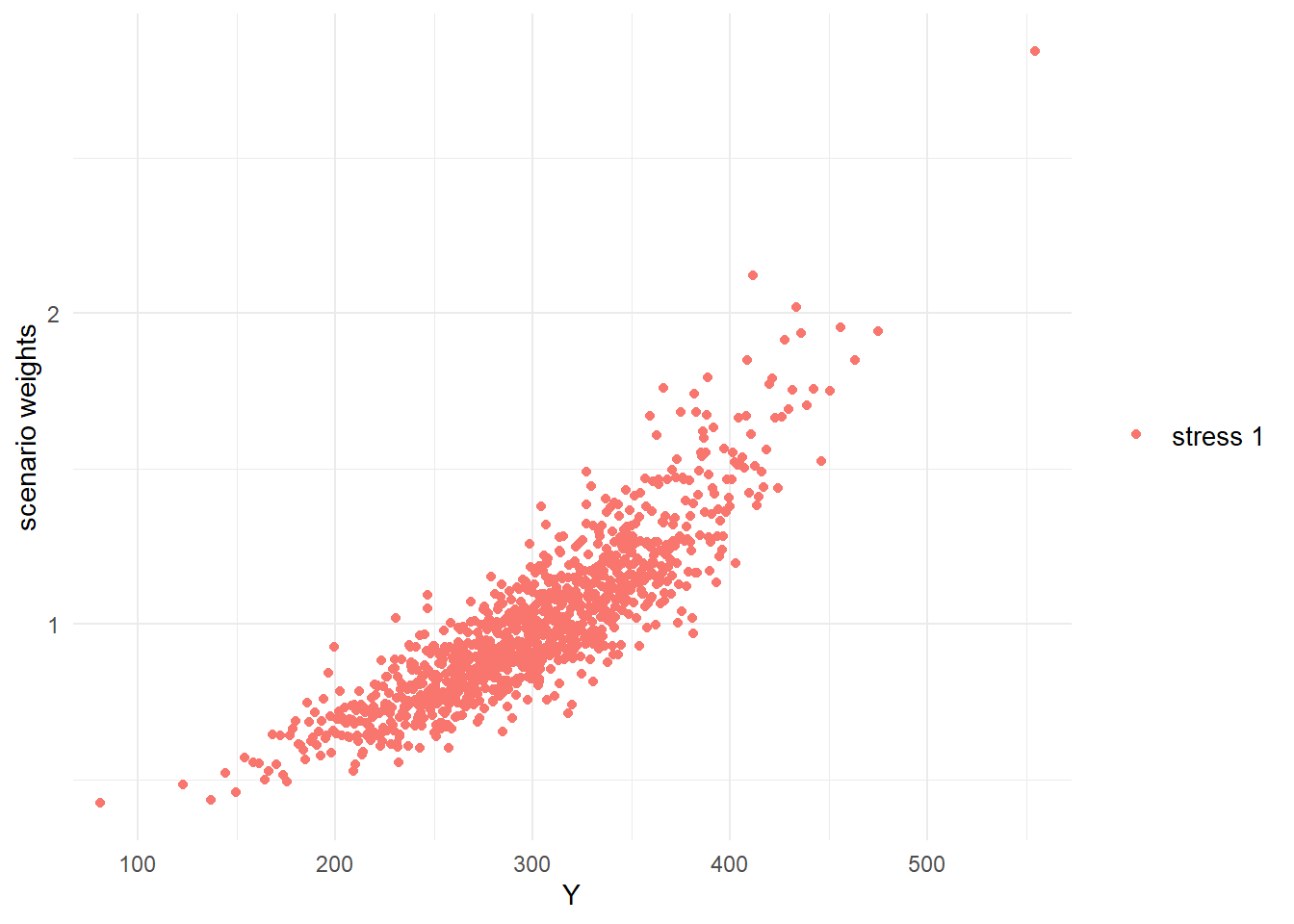Figure 2.2: Scenario weights against observations of model components $$Z_1$$ (left) and $$Y$$ (right), subject to a stress on the mean of $$Z_1$$.

The stress to the mean of $$Z_1$$ did not impact the volatility of either $$Z_1$$ or $$Y$$, as can be seen by the practically unchanged standard deviations in the output of summary(str.mean). Thus, we introduce an alternative stress that keeps the mean of $$Z_1$$ fixed at 100, but increases its standard deviation from 40 to 50. This new stress is seen to impact the standard deviation of the portfolio loss $$Y$$.

str.sd <- stress(type = "mean sd", x = dat, k = 1, new_means = 100, new_sd = 50)
##   cols required_moment achieved_moment abs_error rel_error
## 1    1             100             100   3.0e-07   3.0e-09
## 2    1           12500           12500   5.2e-05   4.2e-09
summary(str.sd, base = FALSE)
## $stress 1 ## Z1 Z2 Z3 Y ## mean 100.0000 99.941 99.9782 299.9187 ## sd 50.0002 21.349 19.9799 67.9230 ## skewness -0.0027 0.007 -0.0034 0.0049 ## ex kurtosis -0.0556 -0.033 -0.0061 -0.0426 ## 1st Qu. 66.0964 85.495 86.4822 253.7496 ## Median 100.1290 99.974 100.0455 299.9766 ## 3rd Qu. 133.7733 114.301 113.4701 345.9159 Furthermore, in Figure 2.3, we compare the baseline and stressed cdfs of $$Z_1$$ and $$Y$$, under the new stress on $$Z_1$$. The crossing of probability distribution reflects the increase in volatility. plot_cdf(str.sd, xCol = "Z1", base = TRUE) plot_cdf(str.sd, xCol = 4, base = TRUE)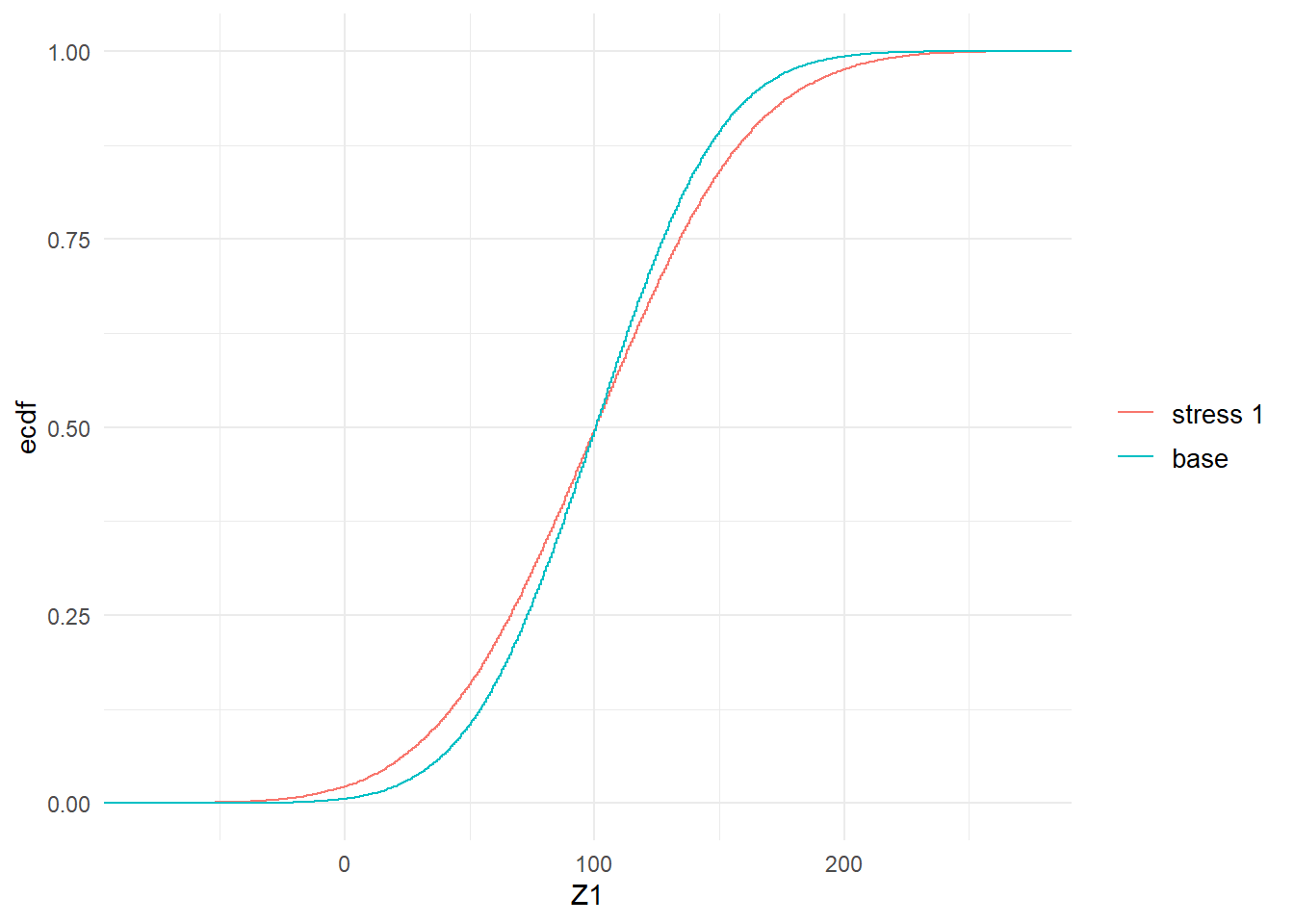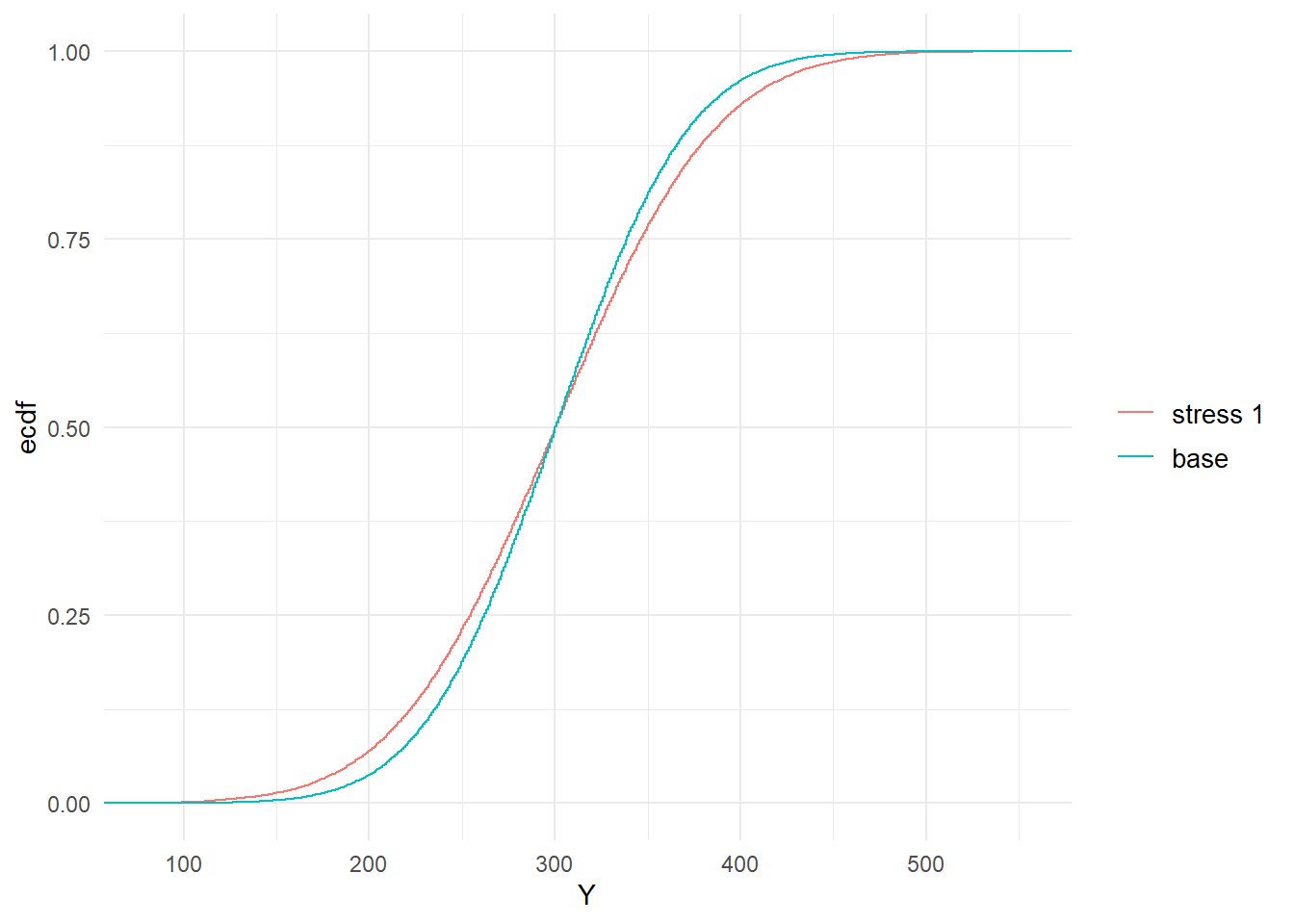Figure 2.3: Baseline and stressed empirical distribution functions of model components $$Z_1$$ (left) and $$Y$$ (right), subject to a stress on the standard deviation of $$Z_1$$. The different way in which a stress on the standard deviation of $$Z_1$$ impacts on the model, compared to a stress on the mean, is reflected by the scenario weights. Figure 2.4 shows the pattern of the scenario weights and how, when stressing standard deviations, higher weight is placed on scenarios where $$Z_1$$ is extreme, either much lower or much higher than its mean of 100. plot_weights(str.sd, xCol = "Z1", n = 2000) plot_weights(str.sd, xCol = "Y", n = 2000)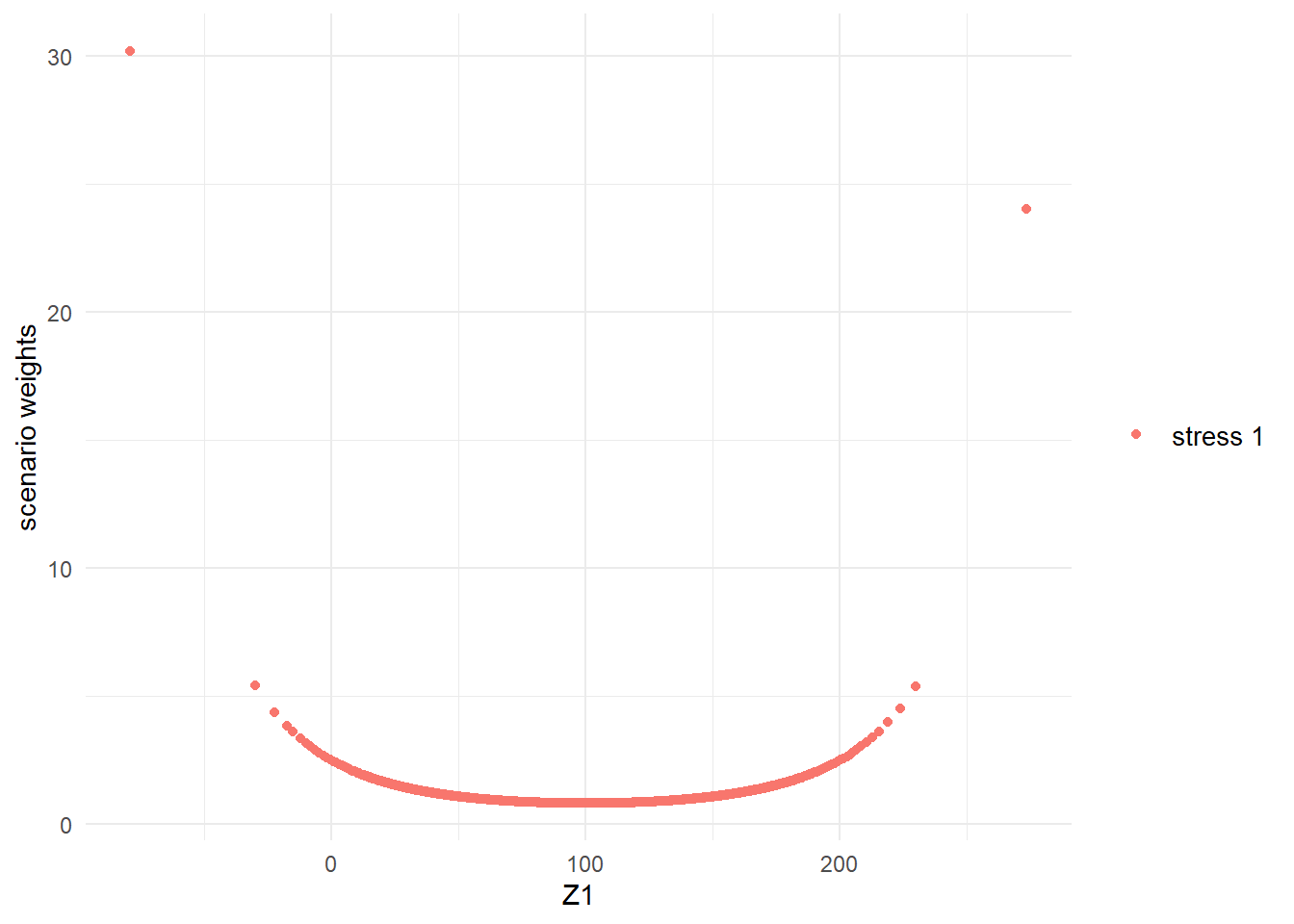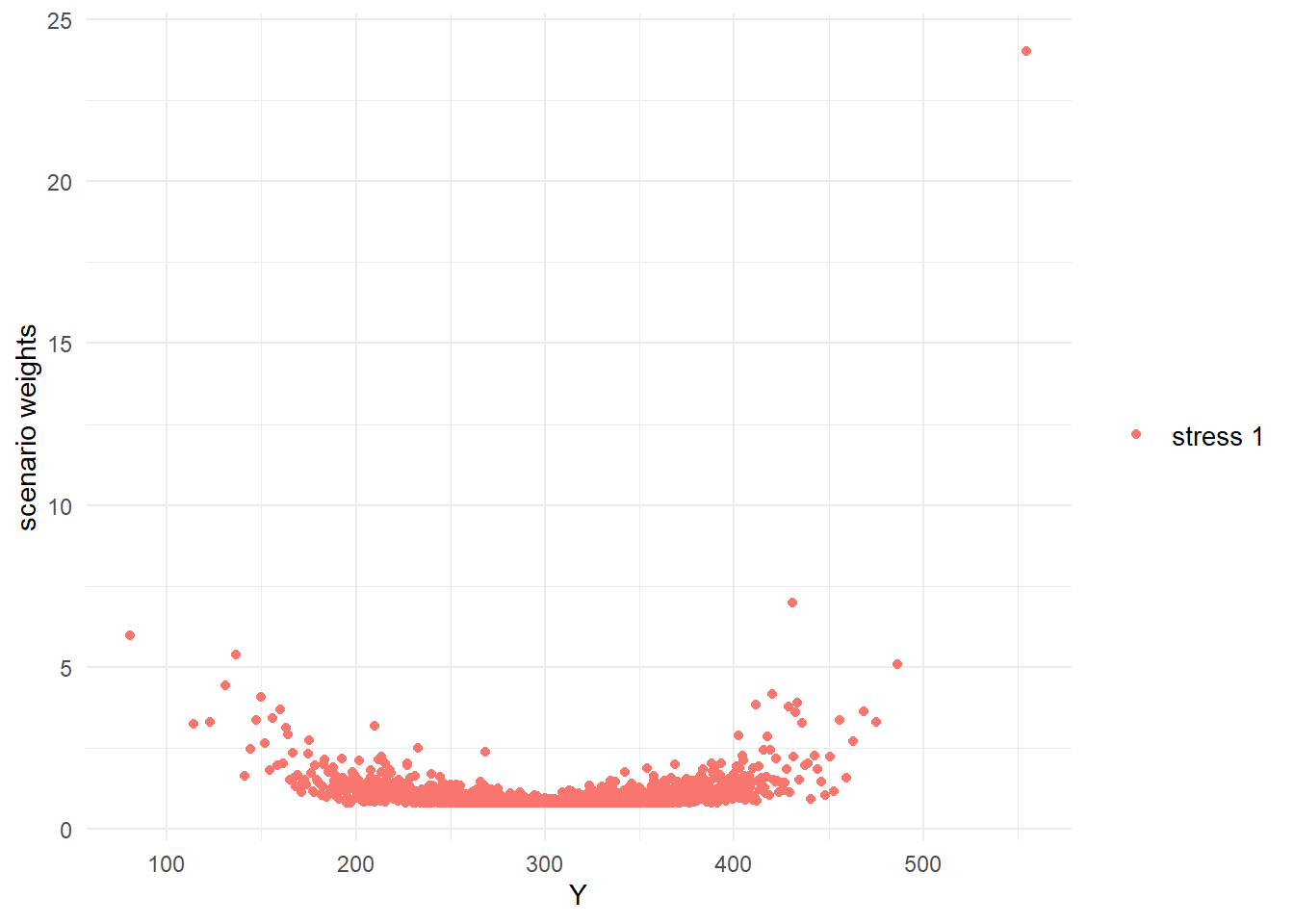Figure 2.4: Scenario weights against observations of model components $$Z_1$$ (left) and $$Y$$ (right), subject to a stress on the standard deviation of $$Z_1$$. Finally we ought to note that not all stresses that one may wish to apply are feasible. Assume for example that we want to increase the mean of $$Z_1$$ from 100 to 300, which exceeds the maximum realisation of $$Z_1$$ in the baseline model. Then, clearly, no set of scenario weights can be found that produce a stress that yields the required mean for $$Z_1$$; consequently an error message is produced. stress(type = "mean", x = dat, k = 1, new_means = 300) ## Error in stress_moment(x = x, f = means, k = as.list(k), m = new_means, : Values in m must be in the range of f(x) max(Z1) ##  273 # 3 Scope of the SWIM package ## 3.1 Stressing a model We briefly introduce key concepts, using slightly more technical language compared to Section 2. A model consists of a random vector of model components $$\mathbf X = (X_1,\dots,X_d)$$ and a probability measure; we denote the probability measure of a baseline model by $$P$$ and that of a stressed model by $$P^W$$, where $$W= \frac{dP^W}{dP}$$, satisfying $$E(W)=1$$ and $$W\geq 0$$, is a Radon-Nikodym derivative. In a Monte Carlo simulation context, the probability space is discrete with $$n$$ states $$\Omega=\{\omega_1,\dots,\omega_n\}$$, each of which corresponds to a simulated scenario. To reconcile this formulation with the notation of Section 2, we denote, for $$i=1, \dots, n,~j=1,\dots, d$$, the realisations $$X_j(\omega_i):= x_{ji}$$ and $$W(\omega_i):=w_i$$; the latter are the scenario weights. Under the baseline model, each scenario has the same probability $$P(\omega_i)=1/n$$, while under a stressed model it is $$P^W(\omega_i)=W(\omega_i)/n=w_i/n$$. The stressed model thus arises from a change of measure from $$P$$ to $$P^W$$, which entails the application of scenario weights $$w_1,\dots, w_n$$ on individual simulations. SWIM calculates scenario weights such that model components fulfil specific stresses, while the distortion to the baseline model is as small as possible when measured by the Kullback-Leibler divergence (or relative entropy). Mathematically, a stressed model is derived by solving $\begin{equation} \min_{ W } ~E(W \log (W)), \quad \text{subject to constraints on } \mathbf X \text{ under } P^W. \tag{3.1} \end{equation}$ In what follows, we denote by a superscript $$W$$ quantities of interest under the stressed model, such as $$F^W, ~ E^W$$ for the probability distribution and expectation under the stressed model, respectively. We refer to and references therein for further mathematical details and derivations of solutions to (3.1). Table 3.1 provides a collection of all implemented types of stresses in the SWIM package. The precise constraints of (3.1) are explained below. Table 3.1: Implemented types of stresses in SWIM. R function Stress type Reference stress wrapper for the stress_type functions Sec. 3.1.1 stress_user user defined scenario weights user Sec. 3.1.5 stress_prob probabilities of disjoint intervals prob Eq. (3.2) stress_mean means mean Eq. (3.3) stress_mean_sd means and standard deviations mean sd Eq. (3.4) stress_moment moments (of functions) moment Eq. (3.5) stress_VaR VaR risk measure (quantile) VaR Eq. (3.6) stress_VaR_ES VaR and ES risk measures VaR ES Eq. (3.7) The solutions to the optimisations (3.2) and (3.6) are worked out fully analytically , whereas problems (3.3), (3.4), (3.5) and (3.7) require some root-finding. Specifically, problems (3.3), (3.4) and (3.5) rely on the package nleqslv, whereas (3.7) uses the uniroot function. ### 3.1.1 The stress function and the SWIM object The stress function is a wrapper for the stress_type functions, where stress(type = "type", ) and stress_type are equivalent. The stress function solves optimisation (3.1) for constraints specified through type and returns a SWIM object, that is, a list including the elements shown in Table 3.2: Table 3.2: The SWIM object, returned by any stress function. R output Description x realisations of the model new_weights scenario weights type type of stress specs details about the stress The data frame containing the realisations of the baseline model, x in the above table, can be extracted from a SWIM object using get_data. Similarly, get_weights and get_weightsfun provide the scenario weights, respectively the functions that, when applied to x, generate the scenario weights. The details of the applied stress can be obtained using get_specs. ### 3.1.2 Stressing disjoint probability intervals Stressing probabilities of disjoint intervals allows defining stresses by altering the probabilities of events pertaining to a model component. The scenario weights are calculated via stress_prob, or equivalently stress(type = "prob", ), and the disjoint intervals are specified through the lower and upper arguments, the endpoints of the intervals. Specifically, stress_prob solves (3.1) with the constraints $\begin{equation} P^W(X_j \in B_k) = \alpha_k, ~k = 1, \ldots, K, \tag{3.2} \end{equation}$ for disjoint intervals $$B_1, \ldots, B_K$$ with $$P(X_j \in B_k) >0$$ for all $$k = 1, \ldots, K$$, and $$\alpha_1, \ldots, \alpha_K > 0$$ such that $$\alpha_1 + \ldots + \alpha_K \leq 1$$ and a model component $$X_j$$. ### 3.1.3 Stressing moments The functions stress_mean, stress_mean_sd and stress_moment provide stressed models with moment constraints. The function stress_mean returns a stressed model that fulfils constraints on the first moment of model components. Specifically, stress_mean solves (3.1) with the constraints $\begin{equation} E^W(X_j) = m_j, ~j \in J, \tag{3.3} \end{equation}$ for $$m_j, ~ j \in J$$, where $$J$$ is a subset of $$\{1, \ldots, d\}$$. The arguments $$m_j$$ are specified in the stress_mean function through the argument new_means. The stress_mean_sd function allows to stress simultaneously the mean and the standard deviation of model components. Specifically, stress_mean_sd solves (3.1) with the constraints $\begin{equation} E^W(X_j) = m_j \text{ and Var}^W(X_j) = s_j^2 , ~j \in J, \tag{3.4} \end{equation}$ for $$m_j, s_j, ~ j \in J$$, where $$J$$ is a subset of $$\{1, \ldots, d\}$$. The arguments $$m_j, s_j$$ are defined in the stress_mean_sd function by the arguments new_means and new_sd respectively. The functions stress_mean and stress_mean_sd are special cases of the general stress_moment function, which allows for stressed models with constraints on functions of the (joint) moments of model components. Specifically For $$k = 1, \ldots, K$$, $$J_k$$ subsets of $$\{1, \ldots, d\}$$ and functions $$f_k \colon \mathbb{R}^{|J_k|} \to \mathbb{R}$$, stress_moment solves (3.1) with the constraints $\begin{equation} E^W(f_k(\mathbf X_{J_k}) ) = m_k, ~k = 1, \ldots, K, \tag{3.5} \end{equation}$ for $$m_k, ~k=1, \dots,K$$ and $$\mathbf X_{J_k}$$ the subvector of model components with indices in $$J_k$$. Note that stress_moment not only allows to define constraints on higher moments of model components, but also to construct constraints that apply to multiple model components simultaneously. For example, the stress $$E^W(X_h X_l) =m_k$$ is achieved by setting $$f_k(x_h, x_l) = x_h x_l$$ in (3.5) above. The functions stress_mean, stress_mean_sd and stress_moment can be applied to multiple model components and are the only stress functions that have scenario weights calculated via numerical optimisation, using the nleqslv package. Thus, depending on the choice of constraints, existence or uniqueness of a stressed model is not guaranteed. ### 3.1.4 Stressing risk measures The functions stress_VaR and stress_VaR_ES provide stressed models, under which a model component fulfils a stress on the risk measures Value-at-Risk ($$\text{VaR}$$) and/or Expected Shortfall ($$\text{ES}$$). The $$\text{VaR}$$ at level $$0 < \alpha < 1$$ of a random variable $$Z$$ with distribution $$F$$ is defined as its left-inverse evaluated at $$\alpha$$, that is $\text{VaR}_\alpha(Z) = F^{-1}(\alpha) = \inf\{ y \in \mathbb{R} ~|~F(y) \geq \alpha\}.$ The $$\text{ES}$$ at level $$0 < \alpha < 1$$ of a random variable $$Z$$ is given by $\text{ES}_\alpha(Z) =\frac{1}{1-\alpha}\int_\alpha^1 \text{VaR}_u(Z) \mathrm{d}u.$ The details of the constraints that stress_VaR and stress_VaR_ES solve, are as follows: For $$0< \alpha <1$$ and $$q, s$$ such that $$q < s$$, stress_VaR solves (3.1) with the constraint $\begin{equation} \text{VaR}_{\alpha }^W(X_j) = q; \tag{3.6} \end{equation}$ and stress_VaR_ES solves (3.1) with the constraints $\begin{equation} \text{VaR}_{\alpha }^W(X_j) = q \text{ and ES}_{\alpha }^W(X_j) = s.\tag{3.7} \end{equation}$ Note that, since SWIM works with discrete distributions, the exact required VaR may not be achievable. In that case, stress_VaR will return scenario weights inducing the largest quantile in the dataset smaller or equal to the required VaR (i.e. $$q$$); this guarantees that $$P^W(X_j\leq q)=\alpha$$. ### 3.1.5 User defined scenario weights The option type = "user" allows to generate a SWIM object with scenario weights defined by a user. The scenario weights can be provided directly via the new_weights argument or through a list of functions, new_weightsfun, that applied to the data x generates the scenario weights. ## 3.2 Analysis of stressed models Table 3.3 provides a complete list of all implemented R functions in SWIM for analysing stressed models, which are described below in detail. Table 3.3: Implemented R function in SWIM for analysing stressed models. R function Analysis of stressed models summary summary statistics cdf cumulative distribution function quantile_stressed quantile function VaR_stressed VaR ES_stressed ES sensitivity sensitivity measures importance_rank importance ranks plot_cdf plots cumulative distributions functions plot_quantile plots quantile functions plot_weights plots scenario weights plot_hist plots histograms plot_sensitivity plots sensitivity measures ### 3.2.1 Distributional comparison The SWIM package contains functions to compare the distribution of model components under different (stressed) models. The function summary is a method for an object of class SWIM and provides summary statistics of the baseline and stressed models. If the SWIM object contains more than one set of scenario weights, each corresponding to one stressed model, the summary function returns for each set of scenario weights a list, containing the elements shown in Table 3.4. Table 3.4: The output of the summary function applied to a SWIM object. R output Description mean sample mean sd sample standard deviation skewness sample skewness ex kurtosis sample excess kurtosis 1st Qu. $$25%$$ quantile Median median, $$50%$$ quantile 3rd Qu. $$75%$$ quantile The empirical distribution function of model components under a stressed model2 can be calculated using the cdf function of the SWIM package, applied to a SWIM object. To calculate sample quantiles of stressed model components, the function quantile_stressed can be used. The function VaR_stressed and ES_stressed provide the stressed VaR and ES of model components, which is of particular interest for stressed models resulting from constraints on risk measures, see Section 3.1.4. (While quantile_stressed works very similarly to the base R function quantile, VaR_stressed provides better capabilities for comparing different models and model components.) Implemented visualisation of distribution functions are plot_cdf, for plotting empirical distribution functions, and plot_hist, for plotting histograms of model components under different (stressed) models. ### 3.2.2 Sensitivity measures Comparison of baseline and stressed models and how model components change under different models, is typically done via sensitivity measures. The SWIM packages contains the sensitivity function, which calculates sensitivity measures of stressed models and model components. The implemented sensitivity measures, summarised in the table below, are the Wasserstein, Kolmogorov and the Gamma sensitivity measures, see . Table 3.5: Definition of the sensitivity measures implemented in SWIM. Metric Definition Wasserstein $$\int | F^W_X (x) - F_X(x)| dx$$ Kolmogorov $$\sup_x |F^W_X (x) - F_X(x)|$$ Gamma $$\frac{E^W(X) - E(X)}{c}$$, for a normalisation $$c$$ The Gamma sensitivity measure is normalised such that it takes values between -1 and 1, with higher positive (negative) values corresponding to a larger positive (negative) impact of the stress on the particular model component. The sensitivity measures can be plotted using plot_sensitivity. The function importance_rank returns the effective rank of model components according to the chosen sensitivity measure. # 4 Case study ## 4.1 A credit risk portfolio The credit model in this section is a conditionally binomial loan portfolio model including systematic and specific portfolio risk. We refer to the Appendix A for details about the model and the generation of the simulated data. A key variable of interest is the total aggregate portfolio loss $$L = L_1 + L_2 + L_3$$, where $$L_1, L_2, L_3$$ are homogeneous subportfolios on a comparable scale (say, thousands of$). The dataset contains 100,000 simulations of the portfolio $$L$$, the subportfolios $$L_1, L_2, L_3$$ as well as the random default probabilities within each subportfolio, $$H_1, H_2, H_3$$. These default probabilities represent the systematic risk within each subportfolio, while their dependence structure represents a systematic risk effect between the subportfolios. The simulated data of the credit risk portfolio are included in the SWIM package and can be accessed via data("credit_data"). A snippet of the dataset looks as follows:

data("credit_data")
head(credit_data)
##         L L1    L2  L3       H1      H2     H3
## [1,]  692  0 346.9 345 1.24e-04 0.00780 0.0294
## [2,] 1006 60 515.6 430 1.16e-03 0.01085 0.0316
## [3,] 1661  0 806.2 855 5.24e-04 0.01490 0.0662
## [4,] 1708  0 937.5 770 2.58e-04 0.02063 0.0646
## [5,]  807  0  46.9 760 8.06e-05 0.00128 0.0632
## [6,] 1159 20 393.8 745 2.73e-04 0.00934 0.0721

## 4.2 Stressing the portfolio loss

In this section, following a reverse sensitivity approach, we study the effects that stresses on (the tail of) the aggregate portfolio loss $$L$$ have on the three subportfolios; thus assessing their comparative importance. First, we impose a $$20\%$$ increase on the VaR at level $$90\%$$ of the portfolio loss.

stress.credit <- stress(type = "VaR", x = credit_data, k = "L", alpha = 0.9,
q_ratio = 1.2)
## Stressed VaR specified was 2174.25 , stressed VaR achieved is 2173.75

The $$20\%$$ increase was specified by setting the q_ratio argument to $$1.2$$ – alternatively the argument q can be set to the actual value of the stressed VaR.

Using the function VaR_stressed, we can quantify how tail quantiles of the aggregate portfolio loss change, when moving from the baseline to the stressed model. We observe that the increase in the VaR of the portfolio loss changes more broadly its tail quantiles; thus the stress on VaR also induces an increase in ES. The implemented functions VaR_stressed and ES_stressed calculate respectively VaR and ES; the argument alpha specifies the levels of VaR and ES, respectively, while the stressed model under which the risk measures are calculated can be chosen using wCol (by default equal to 1).

VaR_stressed(object = stress.credit, alpha = c(0.75, 0.9, 0.95, 0.99),
xCol = "L", wCol = 1, base = TRUE)
##        L base L
## 75% 1506   1399
## 90% 2174   1812
## 95% 2426   2085
## 99% 2997   2671
ES_stressed(object = stress.credit, alpha = 0.9, xCol = "L", wCol = 1,
base = TRUE)
##        L base L
## 90% 2535   2191

As a second stress, we consider, additionally to the $$20\%$$ increase in the $$\text{VaR}_{0.9}$$, an increase in $$\text{ES}_{0.9}$$ of the portfolio loss $$L$$. When stressing VaR and ES together via stress_VaR_ES, both VaR and ES need to be stressed at the same level, here alpha = 0.9. We observe that when stressing the VaR alone, ES increases to 2535. For the second stress we want a greater impact on ES, thus we require that the stressed ES be equal to 3500. This can be achieved by specifying the argument s, which is the stressed value of ES (rather than s_ratio, the proportional increase).

stress.credit <- stress(type = "VaR ES", x = stress.credit, k = "L", alpha = 0.9,
q_ratio = 1.2, s = 3500)
## Stressed VaR specified was 2174.25 , stressed VaR achieved is 2173.75

When applying the stress function or one of its alternative versions to a SWIM object rather than to a data frame (via x = stress.credit in the example above), the result will be a new SWIM object with the new stress “appended” to existing stresses. This is convenient when large datasets are involved, as the stress function returns an object containing the original simulated data and the scenario weights. Note however, that this only works if the underlying data are exactly the same.

## 4.3 Analysing stressed models

The summary function provides a statistical summary of the stressed models. Choosing base = TRUE compares the stressed models with the the baseline model.

summary(stress.credit, base = TRUE)
## $base ## L L1 L2 L3 H1 H2 H3 ## mean 1102.914 19.96 454.04 628.912 0.000401 0.00968 0.0503 ## sd 526.536 28.19 310.99 319.713 0.000400 0.00649 0.0252 ## skewness 0.942 2.10 1.31 0.945 1.969569 1.30836 0.9501 ## ex kurtosis 1.326 6.21 2.52 1.256 5.616080 2.49803 1.2709 ## 1st Qu. 718.750 0.00 225.00 395.000 0.000115 0.00490 0.0318 ## Median 1020.625 0.00 384.38 580.000 0.000279 0.00829 0.0464 ## 3rd Qu. 1398.750 20.00 609.38 810.000 0.000555 0.01296 0.0643 ## ##$stress 1
##                   L    L1     L2     L3       H1      H2     H3
## mean        1193.39 20.83 501.10 671.46 0.000417 0.01066 0.0536
## sd           623.48 29.09 363.57 361.21 0.000415 0.00756 0.0285
## skewness       1.01  2.09   1.36   1.02 1.973367 1.35077 1.0284
## ex kurtosis    0.94  6.14   2.24   1.22 5.630325 2.23363 1.2383
## 1st Qu.      739.38  0.00 234.38 405.00 0.000120 0.00512 0.0328
## Median      1065.62 20.00 412.50 605.00 0.000290 0.00878 0.0483
## 3rd Qu.     1505.62 40.00 675.00 865.00 0.000578 0.01422 0.0688
##
## \$stress 2
##                   L    L1     L2     L3       H1      H2     H3
## mean        1289.90 21.70 558.27 709.93 0.000437 0.01180 0.0566
## sd           875.89 30.57 507.78 447.30 0.000448 0.01045 0.0351
## skewness       1.90  2.17   2.10   1.57 2.090457 2.10131 1.5385
## ex kurtosis    3.67  6.74   4.79   2.80 6.203613 4.97016 2.6143
## 1st Qu.      739.38  0.00 234.38 405.00 0.000123 0.00512 0.0328
## Median      1065.62 20.00 412.50 605.00 0.000297 0.00879 0.0484
## 3rd Qu.     1505.62 40.00 675.00 875.00 0.000594 0.01439 0.0697

Note that stress 1 is the summary output corresponding to the $$20\%$$ increase in the VaR, while stress 2 corresponds to the stress in both VaR and ES. The information on individual stresses can be recovered through the get_specs function, and the actual scenario weights using get_weights. Since the SWIM object stress.credit contains two stresses, the scenario weights that are returned by get_weights form a data frame consisting of two columns, corresponding to stress 1 and to stress 2, respectively.

get_specs(stress.credit)
##            type k alpha       q    s
## stress 1    VaR L   0.9 2173.75 <NA>
## stress 2 VaR ES L   0.9 2173.75 3500

Next, we produce a scatter plot of the scenario weights against the portfolio loss $$L$$. As the number of scenario weights is large, we only $$5000$$ data points. This can be achieved via the parameter n in the function plot_weights, that has a default of $$n = 5000$$.

plot_weights(stress.credit, xCol = "L", wCol = 1, n = 2000)
# parameter wCol specifies the stresses, whose scenario weights are plotted.
plot_weights(stress.credit, xCol = "L", wCol = 2, n = 7000)